## Emotion Detection from Text

By: Ram on Nov 22, 2020

Emotion is a complex state of feeling that influences physical and psychological changes.  Emotions can be expressed verbally (through words, emojis, or speech - tone of voice) or by using nonverbal expressions such as facial expressions.

There are different models to define the type of emotions. 6 basic type of emotions are: happiness, sadness, disgust, fear, surprise, and anger

In this blog, we aim to detect emotions from tweets using Deep Learning Model.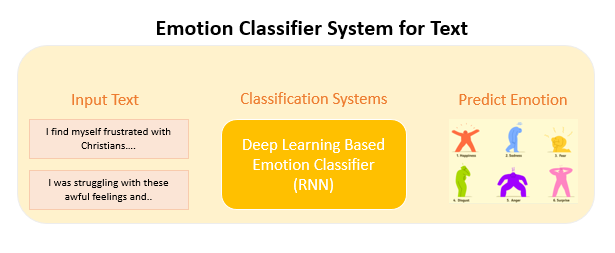### Overview and Data

We have a dataset that has tweets labeled into 6 categories of emotions - Joy, Sadness, Fear, Anger, Love, and Surprise. Source

In this blog, we will build a bidirectional deep neural network to classify the tweets into these emotion categories.

### Helper functions

`import pickle`

`def convert_to_pickle(item, directory):`

`    pickle.dump(item, open(directory,"wb"))`

`def load_from_pickle(directory):`

`    return pickle.load(open(directory,"rb"))`

`# load data`

`data = load_from_pickle(directory="/content/drive/My Drive/MICA/Emotion Detection/tweets.pkl")`

`data.emotions.value_counts().plot.bar()`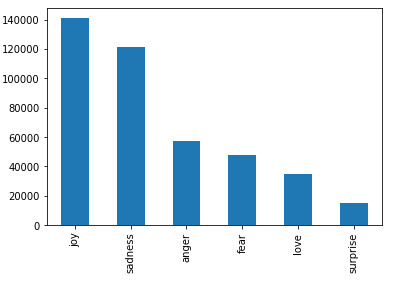### Data Explorations

`data.head()`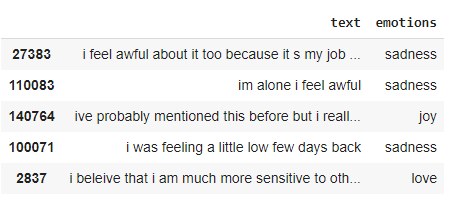Counts of tweets in the data

`len(data)`

Distribution of words counts

`# Tweet Word Counts`

`data['count'] = data['text'].str.count(' ') + 1`

`# Plot `

`import matplotlib.pyplot as plt`

`plt.hist(data['count'], bins=60)`

`plt.title('Word Count Distributions')`

`plt.xlabel('# of Words')`

`plt.ylabel('Freuqnecy')`

`plt.grid(True)`

`plt.show()`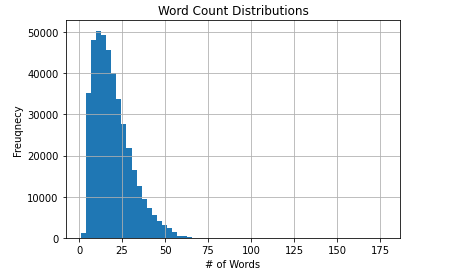### Tokenizer and Word Dictionary

`from keras.preprocessing.text import Tokenizer`

`tokenizer = Tokenizer()`

`tokenizer.fit_on_texts( data['text'])`

Word dictionary length and sample words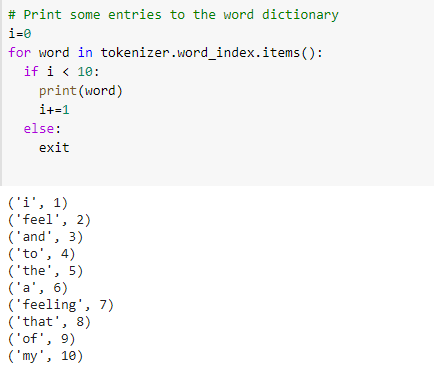### Encode Tweets

Now, we can encode the tweet text with the integer values.

`tweet_encoded = tokenizer.texts_to_sequences(data['text'])`

`tweet_encoded[0:5]`

For us to train a recurrent neural network (RNN), input sequences have to be of the same length.

We are padding the sequences. If we look at the distributions, most of the tweets have word counts less than 70. So, we can fix the max sequence length as 70. We will do the right padding-right blank spaces will be added with the word "post".

`import tensorflow as tf`

`# Padding the input and output tensor to the maximum length`

`tweet_tensor = tf.keras.preprocessing.sequence.pad_sequences(tweet_encoded, ``                                                             maxlen=70,``                                                        padding='post')`

`tweet_tensor[0:5]`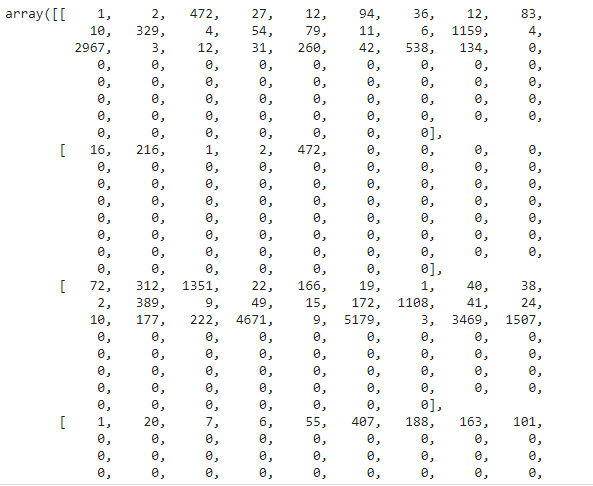### Label Vector

From the input data frame, we will create a label vector.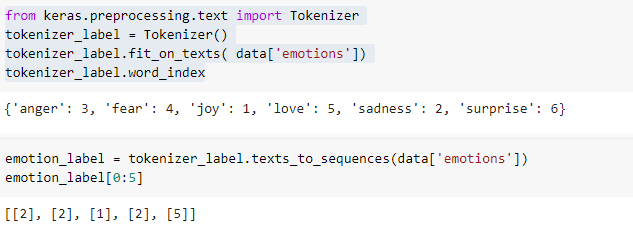### Split Data into Train and Validation Samples

We will split the input data into train and validation samples. Also, we will keep a holdout dataset (test set) for evaluating the models. The sample creation structure is as per the below diagram.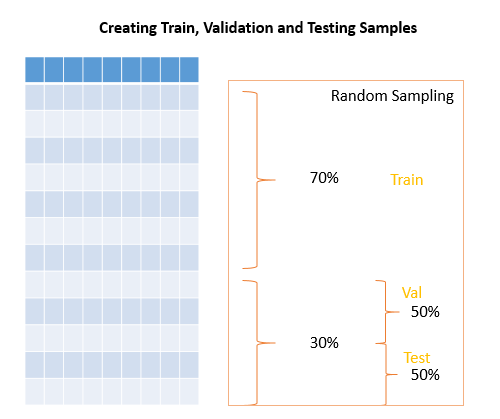`from sklearn.model_selection import train_test_split`

`# Creating training and validation sets using an 70-30 split`

`tweet_tensor_train, tweet_tensor_val, label_tweet_train, label_tweet_val = train_test_split(tweet_tensor, emotion_label, test_size=0.3)`

`# Split the validataion to obtain a holdout sample -- split 50:50`

`tweet_tensor_val, tweet_tensor_test, label_tweet_val, label_tweet_test = train_test_split(tweet_tensor_val, label_tweet_val, test_size=0.5)`

# Show length

`len(tweet_tensor_train), len(label_tweet_train), len(tweet_tensor_val), len(label_tweet_val), len(tweet_tensor_test), len(label_tweet_test)`

`(291766, 291766, 62521, 62521, 62522, 62522)`

### Change Label to One Hot Encoding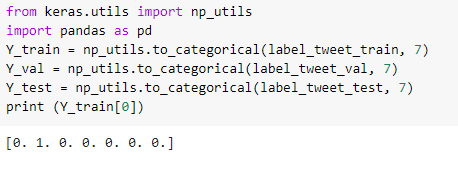### LSTM Recurrent Neural Network

Define model architecture

Embedding - The embedding layer allows to use of the words represented by dense vectors. A word embedding is an approach where a word is presented by a dense vector representation. It has 3 input parameters - input_dim, output_dim and input_length

input_dim = Number of unique words in the input. The embedding layer requires an input sequence as an input to this layer. output_dim = the number of dimensions to embed a word into. Each word is presented by the vector and this is the dimension of that vector. input_length' = length of the maximum document - in this example, we have a fixed size of 2 words

LSTM - Long Short Term Memory Layer: LSTM has a special architecture which enables it to forget the unnecessary information to manage some issues with RNN

`from keras.models import Sequential`

`from keras.layers import Dense`

`from keras.layers import Embedding`

`from keras.layers import Flatten`

`from keras.layers import LSTM`

`# Size of word vector`

`vocab_size = len(tokenizer.word_index) + 1`

`# Define RNN Layers`

`model = tf.keras.models.Sequential()`

`# The Embedding Layer `

`model.add(`

`    tf.keras.layers.Embedding(`

`        input_dim = vocab_size, # The size of our vocabulary `

`        output_dim = 32, # Dimensions to which each words shall be mapped`

`        input_length = 70 # Length of input sequences`

`    )`

`)`

`# Dropout layers: Manage overfitting `

`model.add(`

`    tf.keras.layers.Dropout(`

`        rate=0.25 # Randomly disable 25% of neurons`

`    )`

`)`

`#Optimized LSTM whih is optimised for GPUs. This layer `

`model.add(`

`    tf.compat.v1.keras.layers.LSTM(`

`        units=32 # 32 LSTM units in this layer`

`    )`

`)`

`# Dropout layer `

`model.add(`

`    tf.keras.layers.Dropout(`

`        rate=0.25 # Randomly disable 25% of neurons`

`    )`

`)`

`# Flatten 2D to 1D`

`model.add(Flatten())`

`model.add(Dense(7, activation='softmax'))`

`# Compile the model`

`model.compile(`

`    loss=tf.keras.losses.categorical_crossentropy, # loss function`

`    optimizer=tf.keras.optimizers.Adam(), # optimiser function`

`    metrics=['accuracy']) # reporting metric`

`# Display a summary of the models structure`

`model.summary()`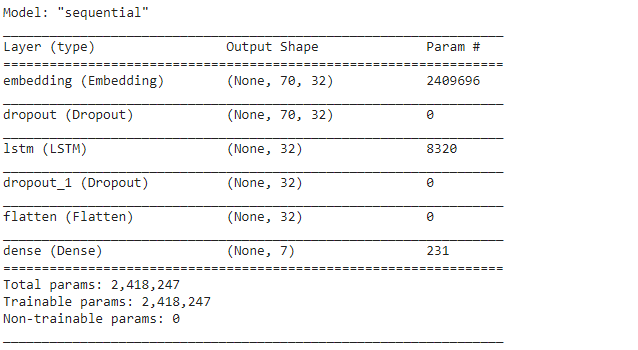### Fit Model

`X_train = tweet_tensor_train.reshape(len(tweet_tensor_train),70,1)`

`X_val = tweet_tensor_val.reshape(len(tweet_tensor_val),70,1)`

`print(X_train.shape)`

`print(X_val.shape)`

`(291766, 70, 1)`

`(62521, 70, 1)`

`# fit network`

`history=model.fit(X_train,`

`                  Y_train,`

`                  epochs=20,`

`                  validation_data = (X_val, Y_val),`

`                  verbose=2)`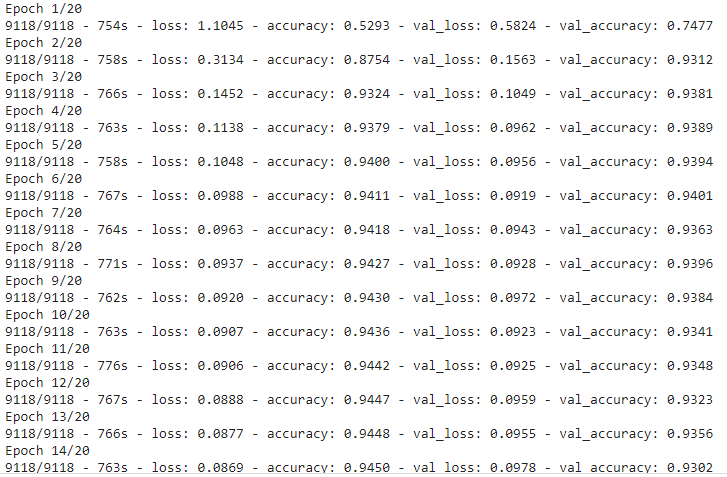### Save Model

`from keras.models import model_from_yaml`

`# serialize model to YAML`

`model_yaml = model.to_yaml()`

`with open("/content/drive/My Drive/MICA/Emotion Detection/model.yaml", "w") as yaml_file:`

`    yaml_file.write(model_yaml)`

`# serialize weights to HDF5`

`model.save_weights("/content/drive/My Drive/MICA/Emotion Detection/model_emotions.h5")`

`Load Model`

`# load YAML and create model`

`yaml_file = open('/content/drive/My Drive/MICA/Emotion Detection/model.yaml', 'r')`

`loaded_model_yaml = yaml_file.read()`

`yaml_file.close()`

`loaded_model = model_from_yaml(loaded_model_yaml)`

`# load weights into new model`

`loaded_model.load_weights("/content/drive/My Drive/MICA/Emotion Detection/model_emotions.h5")`

`print("Loaded model from disk")`

### Model Performance

`# Plot the loss and accuracy curves for training and validation `

`fig, ax = plt.subplots(2,1)`

`ax.plot(history.history['loss'], color='b', label="Training loss")`

`ax.plot(history.history['val_loss'], color='r', label="validation loss",axes =ax)`

`legend = ax.legend(loc='best', shadow=True)`

`ax.plot(history.history['accuracy'], color='b', label="Training accuracy")`

`ax.plot(history.history['val_accuracy'], color='r',label="Validation accuracy")`

`legend = ax.legend(loc='best', shadow=True)`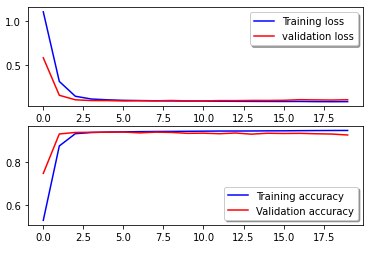### Confusion Matrix

Comparing emotions predicted and the actual label is very important. We can compare using the confusion matrix.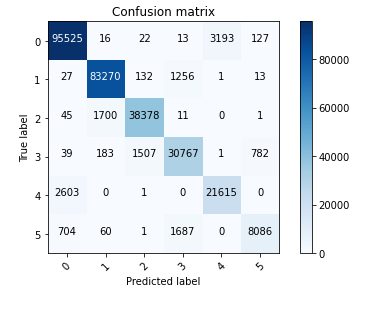As the next steps, we can find ways to improve the model performance. And, once we are happy with the model performance, we can validate the model performance on the test sample (hold out sample).

Tags
Most PopularJun 18, 2020Jul 23, 2020Jun 19, 2020Jun 19, 2021